# HC Verma solutions for Class 11, Class 12 Concepts of Physics Vol. 1 chapter 8 - Work and Energy [Latest edition]

#### Chapters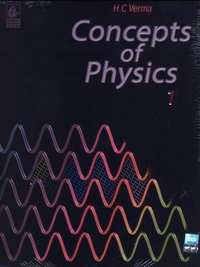## Chapter 8: Work and Energy

Short Answers [Pages 130 - 131]

### HC Verma solutions for Class 11, Class 12 Concepts of Physics Vol. 1 Chapter 8 Work and Energy Short Answers [Pages 130 - 131]

Short Answers | Q 1 | Page 130

When you lift a box from the floor and put it on an almirah the potential energy of the box increases, but there is no change in its kinetic energy. Is it a violation of conservation of energy?

Short Answers | Q 2 | Page 130

A particle is released from the top of an incline of height h. Does the kinetic energy of the particle at the bottom of the incline depend on the angle of incline? Do you need any more information to answer this question in Yes or No?

Short Answers | Q 3 | Page 130

Can the work by kinetic friction on an object be positive? Zero?

Short Answers | Q 4 | Page 130

Can static friction do nonzero work on an object? If yes, give an example. If no, give reason.

Short Answers | Q 5 | Page 130

Can normal force do nonzero work on an object? If yes, give an example. If no, give reason.

Short Answers | Q 6 | Page 130

Can kinetic energy of a system be increased without applying any external force on the system?

Short Answers | Q 7 | Page 130

Is work-energy theorem valid in non-inertial frames?

Short Answers | Q 8 | Page 130

A heavy box is kept on a smooth inclined plane and is pushed up by a force F acting parallel to the plane. Does the work done by the force F as the box goes from A to B depend on how fast the box was moving at A and B? Does the work by the force of gravity depend on this?

Short Answers | Q 9 | Page 130

One person says that the potential energy of a particular book kept in an almirah is 20 J and the other says it is 30 J. One of them necessarily wrong?

Short Answers | Q 10 | Page 130

A book is lifted from the floor and is kept in an almirah. One person says that the potential energy of the book is increased by 20 J and the other says it is increased by 30 J. Is one of them necessarily wrong?

Short Answers | Q 11 | Page 130

In one of the exercises to strengthen the wrist and fingers, a person squeezes and releases a soft rubber ball. Is the work done on the ball positive, negative or zero during compression? During expansion?

Short Answers | Q 12 | Page 130

In tug of war, the team that exerts a larger tangential force on the ground wins. Consider the period in which a team is dragging the opposite team by applying a larger tangential force on the ground. List which of the following works are positive, which are negative and which are zero?

(a) work by the winning team on the losing team
(b) work by the losing team on the winning team
(c) work by the ground on the winning team
(d) work by the ground on the losing team
(e) total external work on the two teams.

Short Answers | Q 13 | Page 131

When an apple falls from a tree what happens to its gravitational potential energy just as it reaches the ground? After it strikes the ground?

Short Answers | Q 14 | Page 131

When you push your bicycle up on an inclined plane, the potential energy of the bicycle and yourself increases. Where does this energy come from?

Short Answers | Q 15 | Page 131

The magnetic force on a charged particle is always perpendicular to its velocity. Can the magnetic force change the velocity of the particles? Speed of the particle?

Short Answers | Q 16.1 | Page 131

A ball is given a speed v on a rough horizontal surface. The ball travels through a distance l on the surface and stops. what are the initial and final kinetic energies of the ball?

Short Answers | Q 16.2 | Page 131

A ball is given a speed v on a rough horizontal surface. The ball travels through a distance l on the surface and stops. What is the work done by the kinetic friction?

Short Answers | Q 17 | Page 131

Consider the situation of the previous question from a frame moving with a speed v0 parallel to the initial velocity of the block. (a) What are the initial and final kinetic energies? (b) What is the work done by the kinetic friction?

MCQ [Page 131]

### HC Verma solutions for Class 11, Class 12 Concepts of Physics Vol. 1 Chapter 8 Work and Energy MCQ [Page 131]

MCQ | Q 1 | Page 131

A heavy stone is thrown from a cliff of height h with a speed v. The stoen will hit the ground with maximum speed if it is thrown

• vertically downward

• vertically upward

• horizontally

• the speed does not depend on the initial direction.

MCQ | Q 2 | Page 131

Two springs A and B(kA = 2kB) are stretched by applying forces of equal magnitudes a the four ends. If the energy stored in A is E, that in B isA

• E/2

•  2E

• E

• E/4

MCQ | Q 3 | Page 131

Two equal masses are attached to the two ends of a spring of spring constant k. The masses are pulled out symmetrically to stretch the spring by a length x over its natural length. The work done by the spring on each mass is

• $\frac{1}{2}\text{kx}^2$

• - $\frac{1}{2}\text{kx}^2$

• $\frac{1}{4}\text{kx}^2$

• - $\frac{1}{4}\text{kx}^2$

MCQ | Q 4 | Page 131

The negative of the work done by the conservative internal forces on a system equal the changes in

• total energy

•  kinetic energy

• potential energy

•  none of these.

MCQ | Q 5 | Page 131

The work done by the external forces on a system equals the change in

•  total energy

• kinetic energy

•  potential energy

• none of these

MCQ | Q 6 | Page 131

The work done by all the forces (external and internal) on a system equals the change in ______.

• total energy

•  kinetic energy

•  potential energy

• none of these

MCQ | Q 7 | Page 131

____________ of a two particle system depends only on the separation between the two particles. The most appropriate choice for the blank space in the above sentence is

• Kinetic energy

•  Total mechanical energy

•  Potential energy

• Total energy.

MCQ | Q 8 | Page 131

A small block of mass m is kept on a rough inclined surface of inclination θ fixed in an elevator. the elevator goes up with a uniform velocity v and the block does not slide on the wedge. The work done by the force of friction on the block in time t will be

• zero

• mgvt cos2θ

• mgvt sin2θ

• mgvt sin 2θ

MCQ | Q 9 | Page 131

A block of mass m slides down a smooth vertical circular track. During the motion, the block is in

• vertical equilibrium

• horizontal equilibrium

•  none of these.

MCQ | Q 10 | Page 131

A particle is rotated in a vertical circle by connecting it to a string of length l and keeping the other end of the string fixed. The minimum speed of the particle when the string is horizontal for which the particle will complete the circle is

• $\sqrt{gl}$

• $\sqrt{2gl}$

• $\sqrt{3gl}$

• $\sqrt{5gl}$

MCQ [Pages 131 - 132]

### HC Verma solutions for Class 11, Class 12 Concepts of Physics Vol. 1 Chapter 8 Work and Energy MCQ [Pages 131 - 132]

MCQ | Q 1 | Page 131

A heavy stone is thrown in from a cliff of height h in a given direction. The speed with which it hits the ground
(a) must depend on the speed of projection
(b) must be larger than the speed of projection
(c) must be independent of the speed of projection
(d) may be smaller than the speed of projection.

MCQ | Q 2 | Page 131

The total work done on a particle is equal to the change in its kinetic energy

• always

• only if the forces acting on it are conservative

• only if gravitational force alone acts on it

• only if elastic force alone acts on it.

MCQ | Q 3 | Page 132

A particle is acted upon by a force of constant magnitude which is always perpendicular to the velocity of the plane. The motion of the particle takes place in a plane. It follows that

(a) its velocity is constant
(b) its acceleration is constant
(c) its kinetic energy is constant
(d) it moves in a circular path.

MCQ | Q 4 | Page 132

Consider two observers moving with respect to each other at a speed v along a straight line. They observe a bock of mass m moving a distance l on a rough surface. The following quantities will be same as observed by the two observers.

• kinetic energy of the block at time t

• work done by friction

• total work done on the block

• acceleration of the block.

MCQ | Q 5 | Page 132

You lift a suitcase from the floor and keep it on a table. The work done by you on the suitcase does not depend on

(a) the path taken by the suitcase
(b) the time taken by you in doing so
(c) the weight of the suitcase

MCQ | Q 6 | Page 132

No work is done by a force on an object if

(a) the force is always perpendicular to its velocity
(b) the force is always perpendicular to its acceleration
(c) the object is stationary but the point of application of the force moves on the object
(d) the object moves in such a way that the point of application of the force remains fixed.

MCQ | Q 7 | Page 132

A particle of mass m is attached to a light string of length l, the other end of which is fixed. Initially the string is kept horizontal and the particle is given an upward velocity v. The particle is just able to complete a circle.
(a) The string becomes slack when the particle reaches its highest point.
(b) The velocity of the particle become zero at the highest point.
(c) The kinetic energy of the ball in initial position was $\frac{1}{2}m v^2 = mgl$

(d) The particle again passes through the initial position.

MCQ | Q 8 | Page 132

The kinetic energy force on the particle continuously increases with time.

(a) The resultant force on the particle must be parallel to the velocity at all instants.
(b) The resultant force on the particle must be at an angle less than 90° all the time.
(c) Its height above the ground level must continuously decrease.
(d) The magnitude of its linear momentum is increasing continuously.

MCQ | Q 9 | Page 132

One end of a light spring of spring constant k is fixed to a wall and the other end is tied to a block placed on a smooth horizontal surface. In a displacement, the work done by the spring is $\frac{1}{2}k x^2$ . The possible cases are

(a) at spring was initially compressed by a distance x and was finally in its natural length
(b) it was initially stretched by a distance x and and finally was in its natural length
(c) it was initially in its natural length and finally in a compressed position
(d) it was initially in its natural length and finally in a stretched position.

MCQ | Q 10 | Page 132

A block of mass M is hanging over a smooth and light pulley through a light string. T he other end of the string is pulled by a constant force F. The kinetic energy of the block increases by 20 J in 1 s.

• The tension in the string is Mg.

• The tension in the string is F.

• The work one by the tension on the block is 20 J in the above 1 s.

• The work done by the force of gravity is −20 J in the above 1 s.

Exercise [Pages 132 - 137]

### HC Verma solutions for Class 11, Class 12 Concepts of Physics Vol. 1 Chapter 8 Work and Energy Exercise [Pages 132 - 137]

Exercise | Q 1 | Page 132

The mass of a cyclist together with the bike is 90 kg. Calculate the increase in kinetic energy if the speed increases from 6⋅0 km/h to 12 km/h.

Exercise | Q 2 | Page 132

A block of mass 2.00 kg moving at a speed of 10.0 m/s accelerates at 3.00 m/s2 for 5.00 s. Compute its final kinetic energy.

Exercise | Q 3 | Page 132

A box is pushed through 4.0 m across a floor offering 100 N resistance. How much work is done by the resisting force?

Exercise | Q 4 | Page 132

A block of mass 5.0 kg slides down an incline of inclination 30° and length 10 m. Find the work done by the force of gravity.

Exercise | Q 5 | Page 132

A constant force of 2⋅5 N accelerates a stationary particle of mass 15 g through a displacement of 2⋅5 m. Find the work done and the average power delivered.

Exercise | Q 6 | Page 132

A particle moves from a point $\overrightarrow{r}_1 = \left( 2 m \right) \overrightarrow{ i } + \left( 3 m \right) \overrightarrow{ j }$ to another point

$\overrightarrow{r}_2 = \left( 3 m \right) \overrightarrow{ i } + \left( 2 m \right) \overrightarrow{ j }$  acts on it. Find the work done by the force on the particle during the displacement.

Exercise | Q 7 | Page 132

A man moves on a straight horizontal road with a block of mass 2 kg in his hand. If he covers a distance of 40 m with an acceleration of 0⋅5 m/s2, find the work done by the man on the block during the motion.

Exercise | Q 8 | Page 133

A force $F = \alpha + bx$  acts on a particle in the x-direction, where a and b are constants. Find the work done by this force during a displacement from x = 0 to x = d.

Exercise | Q 9 | Page 133

A block of mass 250 g slides down an incline of inclination 37° with uniform speed. Find the work done against friction as the block slides through 1m.

Exercise | Q 10 | Page 133

A block of mass m is kept over another block of mass M and the system rests on a horizontal surface (In the following figure). A constant horizontal force F acting on the lower block produces an acceleration $\frac{F}{2 \left( m + M \right)}$   in the system, and the two blocks always move together. (a) Find the coefficient of kinetic friction between the bigger block and the horizontal surface. (b) Find the frictional force acting on the smaller block. (c) Find the work done by the force of friction on the smaller block by the bigger block during a displacement d of the system.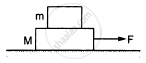Exercise | Q 11 | Page 133

A box weighing 2000 N is to be slowly slid through 20 m on a straight track with friction coefficient 0⋅2 with the box. (a) Find the work done by the person pulling the box with a chain at an angle θ with the horizontal. (b) Find the work when the person has chosen a value of θ, which ensures him the minimum magnitude of the force.

Exercise | Q 12 | Page 133

A block of weight 100 N is slowly moved up a smooth incline of inclination 37° by a person. Calculate the work done by the person in moving the block through a distance of 2 m, if the driving force is (a) parallel to the incline and (b) in the horizontal direction.

Exercise | Q 13 | Page 133

Find the average frictional force needed to stop a car weighing 500 kg at a distance of 25 m if the initial speed is 72 km/h.

Exercise | Q 14 | Page 133

Find the average force needed to accelerate a car weighing 500 kg from rest to 72 km/h through a distance of 25 m.

Exercise | Q 15 | Page 133

A particle of mass m moves on a straight line with its velocity varying with the distance travelled, according to the equation  $\nu = a\sqrt{x}$ , where a is a constant. Find the total work done by all the forces during a displacement from $x = 0 \text{ to } x - d$ .

Exercise | Q 16.1 | Page 133

A block of mass 2 kg kept at rest on an inclined plane of inclination 37° is pulled up the plane by applying a constant force of 20 N parallel to the incline. The force acts for one second.  Show that the work done by the applied force does not exceed 40 J.

Exercise | Q 16.2 | Page 133

A block of mass 2 kg kept at rest on an inclined plane of inclination 37° is pulled up the plane by applying a constant force of 20 N parallel to the incline. The force acts for one second. Find the work done by the force of gravity in that one second if the work done by the applied force is 40 J.

Exercise | Q 16.3 | Page 133

A block of mass 2 kg kept at rest on an inclined plane of inclination 37° is pulled up the plane by applying a constant force of 20 N parallel to the incline. The force acts for one second.  Find the kinetic energy of the block at the instant the force ceases to act. Take g = 10 m/s2.

Exercise | Q 17 | Page 133

A block of mass 2.0 kg is pushed down an inclined plane of inclination 37° with a force of 20 N acting parallel to the incline. It is found that the block moves on the incline with an acceleration of 10 m/s2. If the block started from rest, find the work done (a) by the applied force in the first second, (b) by the weight of the block in the first second and (c) by the frictional force acting on the block in the first second. Take g = 10 m/s2.

Exercise | Q 18 | Page 133

A 250 g block slides on a rough horizontal table. Find the work done by the frictional force in bringing the block to rest if it is initially moving at a speed of 40 cm/s. If the friction coefficient between the table and the block is 0⋅1, how far does the block move before coming to rest?

Exercise | Q 19 | Page 133

Water falling from a 50-m high fall is to be used for generating electric energy. If $1 \cdot 8 \times {10}^5 \text{ kg }$ of water falls per hour and half the gravitational potential energy can be converted into electrical energy, how many 100 W lamps can be lit with the generated energy?

Exercise | Q 20 | Page 133

A person is painting the walls of his house. He stands on a ladder with a bucket containing paint in one hand and a brush in the other. Suddenly the bucket slips from his hand and falls to the floor. If the bucket with the paint had a mass of 6 kg and was at a height of 2 m at the time it slipped, how much gravitational potential energy was lost together with the paint?

Exercise | Q 21 | Page 133

A projectile is fired from the top of a 40 m high cliff with an initial speed of 50 m/s at an unknown angle. Find its speed when it hits the ground.

Exercise | Q 22 | Page 133

The 200 m free-style women's swimming gold medal at Seoul Olympics in 1988 was won by Heike Friendrich of East Germany when she set a new Olympic record of 1 minute and 57⋅56 seconds. Assume that she covered most of the distance with a uniform speed and had to exert 460 W to maintain her speed. Calculate the average force of resistance offered by the water during the swim.

Exercise | Q 23.1 | Page 133

The US athlete Florence Griffith-Joyner won the 100 m sprint gold medal at Seoul Olympics in 1988, setting a new Olympic record of 10⋅54 s. Assume that she achieved her maximum speed in a very short time and then ran the race with that speed till she crossed the line. Take her mass to be 50 kg. Calculate the kinetic energy of Griffith-Joyner at her full speed.

Exercise | Q 23.2 | Page 133

The US athlete Florence Griffith-Joyner won the 100 m sprint gold medal at Seoul Olympics in 1988, setting a new Olympic record of 10⋅54 s. Assume that she achieved her maximum speed in a very short time and then ran the race with that speed till she crossed the line. Take her mass to be 50 kg. Assuming that the track, wind etc. offered an average resistance of one-tenth of her weight, calculate the work done by the resistance during the run.

Exercise | Q 23.3 | Page 133

The US athlete Florence Griffith-Joyner won the 100 m sprint gold medal at Seoul Olympics in 1988, setting a new Olympic record of 10⋅54 s. Assume that she achieved her maximum speed in a very short time and then ran the race with that speed till she crossed the line. Take her mass to be 50 kg.  What power Griffith-Joyner had to exert to maintain uniform speed?

Exercise | Q 24 | Page 133

A water pump lifts water from 10 m below the ground. Water is pumped at a rate of 30 kg/minute with negligible velocity. Calculate the minimum horsepower that the engine should have to do this.

Exercise | Q 25 | Page 133

An unruly demonstrator lifts a stone of mass 200 g from the ground and throws it at his opponent. At the time of projection, the stone is 150 cm above the ground and has a speed of 3 m/s. Calculate the work done by the demonstrator during the process. If it takes one second for the demonstrator to lift the stone and throw it, what horsepower does he use?

Exercise | Q 26 | Page 133

In a factory, 2000 kg of metal needs to be lifted by an engine through a distance of 12 m in 1 minute. Find the minimum horsepower of the engine to be used.

Exercise | Q 27 | Page 133

A scooter company gives the following specifications about its product:
Weight of the scooter − 95 kg
Maximum speed − 60 km/h
Maximum engine power − 3⋅5 hp
Pick up time to get the maximum speed − 5 s
Check the validity of these specifications.

Exercise | Q 28 | Page 134

A block of mass 30 kg is being brought down by a chain. If the block acquires a speed of 40 cm/s in dropping down 2 m, find the work done by the chain during the process.

Exercise | Q 29 | Page 134

The heavier block in an Atwood machine has mass twice that of the lighter one. The tension in the string is 16⋅0 N when the system is set into motion. Find the decrease in the gravitational potential energy during the first second after the system is released from rest.

Exercise | Q 30 | Page 134

The two blocks in an Atwood machine have masses 2 kg and 3 kg. Find the work done by gravity during the fourth second after the system is released from rest.

Exercise | Q 31 | Page 134

Consider the situation shown in the following figure. The system is released from rest and the block of mass 1 kg is found to have a speed 0⋅3 m/s after it has descended a distance of 1 m. Find the coefficient of kinetic friction between the block and the table.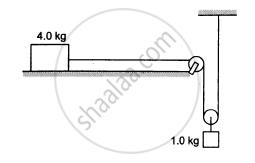Exercise | Q 32 | Page 134

A block of mass 100 g is moved with a speed of 5⋅0 m/s at the highest point in a closed circular tube of radius 10 cm kept in a vertical plane. The cross-section of the tube is such that the block just fits in it. The block makes several oscillations inside the tube and finally stops at the lowest point. Find the work done by the tube on the block during the process.

Exercise | Q 33 | Page 134

A car weighing 1400 kg is moving at a speed of 54 km/h up a hill when the motor stops. If it is just able to reach the destination which is at a height of 10 m above the point, calculate the work done against friction (negative of the work done by friction).

Exercise | Q 34 | Page 134

A small block of mass 200 g is kept at the top of a frictionless incline which is 10 m long and 3⋅2 m high. How much work was required (a) to lift the block from the ground and put it an the top, (b) to slide the block up the incline? What will be the speed of the block when it reaches the ground if (c) it falls off the incline and drops vertically to the ground (d) it slides down the incline? Take g = 10 m/s2

Exercise | Q 35 | Page 134

In a children's park, there is a slide which has a total length of 10 m and a height of 8⋅0 m . A vertical ladder is provided to reach the top. A boy weighing 200 N climbs up the ladder to the top of the slide and slides down to the ground. The average friction offered by the slide is three tenth of his weight. Find (a) the work done by the ladder on the boy as he goes up; (b) the work done by the slide on the boy as he comes down. Neglect any work done by forces inside the body of the boy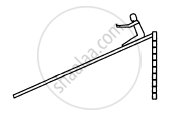Exercise | Q 36 | Page 134

Figure shows a particle sliding on a frictionless track which terminates in a straight horizontal section. If the particle starts slipping from point A, how far away from the track will the particle hit the ground?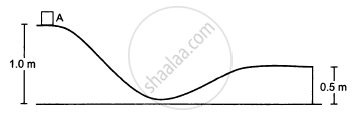Exercise | Q 37 | Page 134

A block weighing 10 N travels down a smooth curved track AB joined to a rough horizontal surface (In the following figure). The rough surface has a friction coefficient of 0⋅20 with the block. If the block starts slipping on the track from a point 1⋅0 m above the horizontal surface, how far will it move on the rough surface?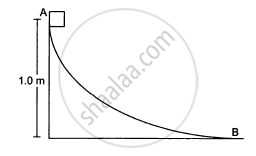Exercise | Q 38 | Page 134

A uniform chain of mass m and length l overhangs a table with its two third part on the table. Find the work to be done by a person to put the hanging part back on the table.

Exercise | Q 39 | Page 134

A uniform chain of length L and mass M overhangs a horizontal table with its two third part on the table. The friction coefficient between the table and the chain is μ . Find the work done by friction during the period the chain slips off the table.

Exercise | Q 40 | Page 134

A block of mass 1 kg is placed at point A of a rough track shown in figure following. If slightly pushed towards right, it stops at point B of the track. Calculate the work done by the frictional force on the block during its transit from A to B.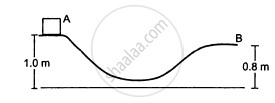Exercise | Q 41 | Page 135

A block of mass 5 kg is suspended from the end of a vertical spring which is stretched by 10 cm under the load of the block. The block is given a sharp impulse from below, so that it acquires an upward speed of 2⋅ m/s. How high will it rise? Take g = 10 m/s2

Exercise | Q 42 | Page 135

A block of mass 250 g is kept on a vertical spring of spring constant 100 N/m fixed from below. The spring is now compressed 10 cm shorter than its natural length and the system is released from this position. How high does the block rise ? Take g = 10 m/s2.

Exercise | Q 43 | Page 135

Following figure shows a spring fixed at the bottom end of an incline of inclination 37°. A small block  of mass 2 kg starts slipping down the incline from a point 4⋅8 m away from the spring. The block compresses the spring by 20 cm, stops momentarily and then rebounds through a distance of 1 m up the incline. Find (a) the friction coefficient between the plane and the block and (b) the spring constant of the spring. Take g = 10 m/s2.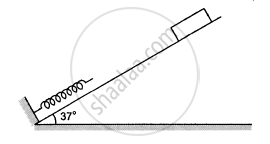Exercise | Q 44 | Page 135

A block of mass m moving at a speed ν compresses a spring through a distance x before its speed is halved. Find the spring constant of the spring.

Exercise | Q 45 | Page 135

Consider the situation shown in the following figure. Initially the spring is unstretched when the system is released from rest. Assuming no friction in the pulley, find the maximum elongation of the spring.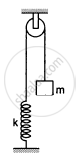Exercise | Q 46 | Page 135

A block of mass m is attached to two unstretched springs of spring constants k1 and k2 as shown in the following figure. The block is displaced towards the right through a distance x and is released. Find the speed of the block as it passes through the mean position shown.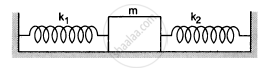Exercise | Q 47 | Page 135

A block of mass m sliding on a smooth horizontal surface with a velocity $\vec{\nu}$ meets a long horizontal spring fixed at one end and with spring constant k, as shown in following figure following. Find the maximum compression of the spring. Will the velocity of the block be the same as  $\vec{\nu}$  when it comes back to the original position shown?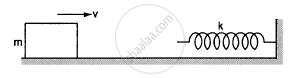Exercise | Q 48 | Page 135

A small block of mass 100 g is pressed against a horizontal spring fixed at one end to compress the spring through 5 cm (figure following). The spring constant is 100 N/m. When released, the block moves horizontally till it leaves the spring. Where will it hit the ground 2 m below the spring?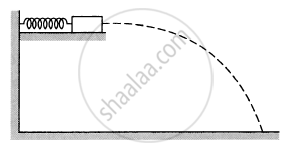Exercise | Q 49 | Page 135

A small heavy block is attached to the lower end of a light rod of length l which can be rotated about its clamped upper end. What minimum horizontal velocity should the block be given so that it moves in a complete vertical circle?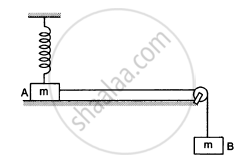Exercise | Q 50 | Page 135

In the following figure shows two blocks A and B, each of mass of 320 g connected by a light string passing over a smooth light pulley. The horizontal surface on which the block Acan slide is smooth. Block A is attached to a spring of spring constant 40 N/m whose other end is fixed to a support 40 cm above the horizontal surface. Initially, the spring is vertical and unstretched when the system is released to move. Find the velocity of the block A at the instant it breaks off the surface below it. Take g = 10 m/s2.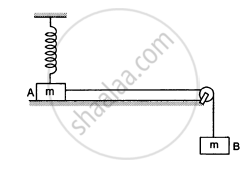Exercise | Q 51 | Page 136

One end of a spring of natural length h and spring constant k is fixed at the ground and the other is fitted with a smooth ring of mass m which is allowed to slide on a horizontal rod fixed at a height h (following figure). Initially, the spring makes an angle of 37° with the vertical when the system is released from rest. Find the speed of the ring when the spring becomes vertical.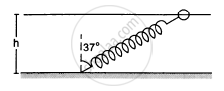Exercise | Q 52 | Page 136

Figure following shows a light rod of length l rigidly attached to a small heavy block at one end and a hook at the other end. The system is released from rest with the rod in a horizontal position. There is a fixed smooth ring at a depth h below the initial position of the hook and the hook gets into the ring as it reaches there. What should be the minimum value of h so that the block moves in a complete circle about the ring?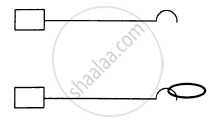Exercise | Q 53 | Page 136

The bob of a pendulum at rest is given a sharp hit to impart a horizontal velocity  $\sqrt{10 \text{ gl }}$, where l is the length of the pendulum. Find the tension in the string when (a) the string is horizontal, (b) the bob is at its highest point and (c) the string makes an angle of 60° with the upward vertical.

Exercise | Q 54 | Page 136

A simple pendulum consists of a 50 cm long string connected to a 100 g ball. The ball is pulled aside so that the string makes an angle of 37° with the vertical and is then released. Find the tension in the string when the bob is at its lowest position.

Exercise | Q 55 | Page 136

Following figure following shows a smooth track, a part of which is a circle of radius R. A block of mass m is pushed against a spring of spring constant k fixed at the left end and is then released. Find the initial compression of the spring so that the block presses the track with a force mg when it reaches the point P, where the radius of the track is horizontal.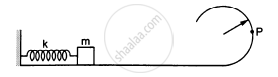Exercise | Q 56 | Page 136

The bob of a stationary pendulum is given a sharp hit to impart it a horizontal speed of $\sqrt{3 gl}$ . Find the angle rotated by the string before it becomes slack.

Exercise | Q 57 | Page 136

A heavy particle is suspended by a 1⋅5 m long string. It is given a horizontal velocity of $\sqrt{57} \text{m/s}$ (a) Find the angle made by the string with the upward vertical when it becomes slack. (b) Find the speed of the particle at this instant. (c) Find the maximum height reached by the particle over the point of suspension. Take g = 10 m/s2

Exercise | Q 58 | Page 136

A simple pendulum of length L with a bob of mass m is deflected from its rest position by an angle θ and released (following figure). The string hits a peg which is fixed at a distance x below the point of suspension and the bob starts going in a circle centred at the peg. (a) Assuming that initially the bob has a height less than the peg, show that the maximum height reached by the bob equals its  initial height. (b) If the pendulum is released with $\theta = 90^\circ \text{ and x = L}/2$ , find the maximum height reached by the bob above its lowest position before the string becomes slack. (c) Find the minimum value of x/L for which the bob goes in a complete circle about the peg when the pendulum is released from $\theta = 90^\circ$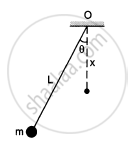Exercise | Q 59 | Page 136

A particle slides on the surface of a fixed smooth sphere starting from the topmost point. Find the angle rotated by the radius through the particle, when it leaves contact with the sphere.

Exercise | Q 60 | Page 136

A particle of mass m is kept on a fixed, smooth sphere of radius R at a position where the radius through the particle makes an angle of 30° with the vertical. The particle is released from this position. (a) What is the force exerted by the sphere on the particle just after the release? (b) Find the distance travelled by the particle before it loses contact with the sphere.

Exercise | Q 61 | Page 136

A particle of mass m is kept on the top of a smooth sphere of radius R. It is given a sharp impulse which imparts it a horizontal speed ν. (a) Find the normal force between the sphere and the particle just after the impulse. (b) What should be the minimum value of ν for which the particle does not slip on the sphere? (c) Assuming the velocity ν to be half the minimum calculated in part, (b) find the angle made by the radius through the particle with the vertical when it leaves the sphere.

Exercise | Q 62.1 | Page 137

Figure ( following ) shows a smooth track which consists of a straight inclined part of length l joining smoothly with the circular part. A particle of mass m is projected up the incline from its bottom. Find the minimum projection-speed $\nu_0$ for which the particle reaches the top of the track.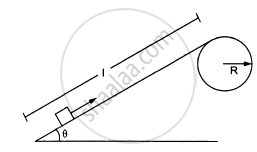Exercise | Q 62.2 | Page 137

Figure ( following ) shows a smooth track which consists of a straight inclined part of length l joining smoothly with the circular part. A particle of mass m is projected up the incline from its bottom. Assuming that the projection-speed is $\nu_0$ and that the block does not lose contact with the track before reaching its top, find the force acting on it when it reaches the top.Exercise | Q 62.3 | Page 137

Figure ( following ) shows a smooth track which consists of a straight inclined part of length l joining smoothly with the circular part. A particle of mass m is projected up the incline from its bottom.Assuming that the projection-speed is only slightly greater than $\nu_0$ , where will the block lose contact with the track?Exercise | Q 63.1 | Page 167

A chain of length l and mass m lies on the surface of a smooth sphere of radius R > l with one end tied to the top of the sphere.  Find the gravitational potential energy of the chain with reference level at the centre of the sphere.

Exercise | Q 63.2 | Page 137

A chain of length l and mass m lies on the surface of a smooth sphere of radius R > l with one end tied to the top of the sphere.  Suppose the chain is released and slides down the sphere. Find the kinetic energy of the chain, when it has slid through an angle θ.

Exercise | Q 63.3 | Page 137

A chain of length l and mass m lies on the surface of a smooth sphere of radius R > l with one end tied to the top of the sphere.  Find the tangential acceleration $\frac{d\nu}{dt}$ of the chain when the chain starts sliding down.

Exercise | Q 64 | Page 137

A smooth sphere of radius R is made to translate in a straight line with a constant acceleration a. A particle kept on the top of the sphere is released at zero velocity with respect to the sphere. Find the speed of the particle with respect to the sphere as a function of the angle θ it slides.

## Chapter 8: Work and Energy## HC Verma solutions for Class 11, Class 12 Concepts of Physics Vol. 1 chapter 8 - Work and Energy

HC Verma solutions for Class 11, Class 12 Concepts of Physics Vol. 1 chapter 8 (Work and Energy) include all questions with solution and detail explanation. This will clear students doubts about any question and improve application skills while preparing for board exams. The detailed, step-by-step solutions will help you understand the concepts better and clear your confusions, if any. Shaalaa.com has the CBSE Class 11, Class 12 Concepts of Physics Vol. 1 solutions in a manner that help students grasp basic concepts better and faster.

Further, we at Shaalaa.com provide such solutions so that students can prepare for written exams. HC Verma textbook solutions can be a core help for self-study and acts as a perfect self-help guidance for students.

Concepts covered in Class 11, Class 12 Concepts of Physics Vol. 1 chapter 8 Work and Energy are Introduction of Work, Energy and Power, Notions of Work and Kinetic Energy: the Work-energy Theorem, Kinetic Energy, Work Done by a Constant Force and a Variable Force, The Concept of Potential Energy, Conservation of Mechanical Energy, Potential Energy of a Spring, Various Forms of Energy : the Law of Conservation of Energy, Non - Conservative Forces - Motion in a Vertical Circle, Concept of Work, Collisions, Power.

Using HC Verma Class 12 solutions Work and Energy exercise by students are an easy way to prepare for the exams, as they involve solutions arranged chapter-wise also page wise. The questions involved in HC Verma Solutions are important questions that can be asked in the final exam. Maximum students of CBSE Class 12 prefer HC Verma Textbook Solutions to score more in exam.

Get the free view of chapter 8 Work and Energy Class 12 extra questions for Class 11, Class 12 Concepts of Physics Vol. 1 and can use Shaalaa.com to keep it handy for your exam preparation